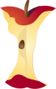Home > Grade Levels > Grade 8 >

## Using Similar Triangles to Find Slope#### Aligned To Common Core Standard:

Grade 8 Number System- 8.EE.B.6

### Printable Worksheets And Lessons

• Slope of Lines Step-by-Step Lesson- Use the triangle method to find the slope of the line. This seems like too much work, but it does give you a definitive answer.

• Guided Lesson - Write an equation for the graphed line and find the slope using the triangle method.

• Guided Lesson Explanation - Number one and three take about a page each to explain. It's good to tackle that skill here.

• Independent Practice - Four full pages of practice work for you here. Equations and slope are all over the place.

• Matching Worksheet - We have line-a-polooza. Tell us the slope or equation of the lines.

###(Click Here to Upgrade)

#### Homework Sheets

Find the slope and equation of a line by using the triangles.

#### Practice Worksheets

All the three standard skills you will see are here.

#### Math Skill Quizzes

Quiz one asks you to find the equation of the line. Quiz two focuses on slope and quiz three goes over all skills.

 Quiz 1 Quiz 2 Quiz 3